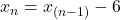## What is a formula for the nth term of the given sequence? -20, -26, -32…

Question

What is a formula for the nth term of the given sequence?
-20, -26, -32…

in progress 0
2 months 2021-07-30T19:08:25+00:00 2 Answers 3 views 0Step-by-step explanation:

b/c

Step-by-step explanation:

the common difference is :

d=-26-(-20)=-6

the nth term is aₙ=a₁+(n-1)d

a₁= -20

aₙ= -20+(n-1)(-6)

aₙ=-20-6n+6=-14-6n